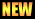# MTH231: Linear Algebra

Linear algebra is the branch of mathematics deals with algebraic equations, spaces (vector and scalar), linear mappings between such spaces etc. Combined with the theory of calculus, linear algebra ensures to have methodologies to compute the solutions of system of equations (algebraic and differential). Techniques from linear algebra are also used in analytically geometry, engineering, physics, natural sciences and computer sciences and particularly in economics. Investigation in linear algebra is initially motivated by a system of linear equations in several unknowns. Such equations are naturally represented using the formalism of matrices and vectors. Linear algebra is central to both pure and applied mathematics.

Main objectives are to introduce the students with this subject, provide basic concepts and terminologies used in it. This course is intended to introduce the concept of system of linear equations and matrices, determinants; vector and inner product spaces, matrix representation of linear transformations, Eigen values and Eigen vectors, Cayley Hamilton theorem and discuss their applications to problems of real life and different field of sciences.

These files are in PDF and it can be only viewed or print if there is PDF reader or viewer installed on you system. See Software section for some PDF reader or viewer.

• Exercise # 3• Elementary Linear Algebra: Applications Version, 11th Edition by Howard Anton and Chris Rorres. (see title on Google books)
• atiq/sp14-mth231
• Last modified: 18 months ago
• (external edit)